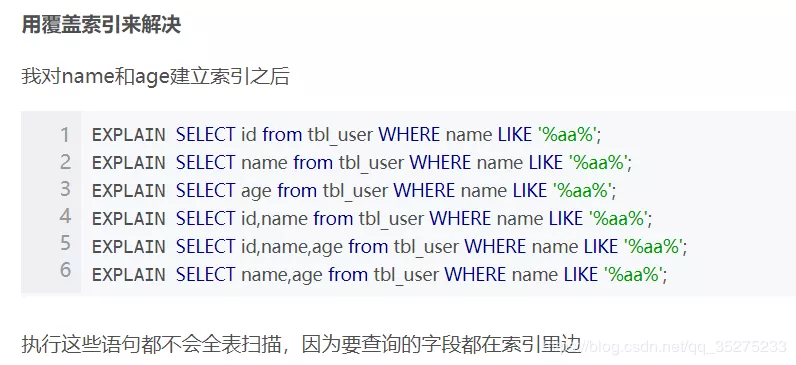# 面試官：談談 MySQL 聯合索引生效、失效的條件？

## 聯合索引失效的條件

``````create table myTest(
a int,
b int,
c int,
KEY a(a,b,c)
);
``````
``````(1) select * from myTest  where a=3 and b=5 and c=4;   ----  abc順序
abc三個索引都在where條件裡面用到了，而且都發揮了作用

(2) select * from myTest  where  c=4 and b=6 and a=3;
where裡面的條件順序在查詢之前會被mysql自動優化，效果跟上一句一樣

(3) select * from myTest  where a=3 and c=7;
a用到索引，b沒有用，所以c是沒有用到索引效果的

(4) select * from myTest  where a=3 and b>7 and c=3;     ---- b範圍值，斷點，阻塞了c的索引
a用到了，b也用到了，c沒有用到，這個地方b是範圍值，也算斷點，只不過自身用到了索引

(5) select * from myTest  where b=3 and c=4;   --- 聯合索引必須按照順序使用，並且需要全部使用

(6) select * from myTest  where a>4 and b=7 and c=9;
a用到了  b沒有使用，c沒有使用

(7) select * from myTest  where a=3 order by b;
a用到了索引，b在結果排序中也用到了索引的效果，a下面任意一段的b是排好序的

(8) select * from myTest  where a=3 order by c;
a用到了索引，但是這個地方c沒有發揮排序效果，因為中間斷點了，使用 explain 可以看到 filesort

(9) select * from mytable where b=3 order by a;
b沒有用到索引，排序中a也沒有發揮索引效果
``````

## 最後說說索引失效的條件

• 不在索引列上做任何操作（計算、函式、（自動or手動）型別轉換），會導致索引失效而轉向全表掃描
• 儲存引擎不能使用索引範圍條件右邊的列
• 儘量使用覆蓋索引（只訪問索引的查詢（索引列和查詢列一致）），減少select *
• mysql在使用不等於（！=或者<>）的時候無法使用索引會導致全表掃描
• is null,is not null也無法使用索引
• like以萬用字元開頭（’%abc…’）mysql索引失效會變成全表掃描的操作。``````SELECT * from staffs where name='2000';
-- 因為mysql會在底層對其進行隱式的型別轉換

SELECT * from staffs where name=2000;
--- 未使用索引
``````

• 對於單鍵索引，儘量選擇針對當前query過濾性更好的索引
• 在選擇組合索引的時候，當前Query中過濾性最好的欄位在索引欄位順序中，位置越靠前越好。
• 在選擇組合索引的時候，儘量選擇可以能夠包含當前query中的where子句中更多欄位的索引
• 儘可能通過分析統計資訊和調整query的寫法來達到選擇合適索引的目的